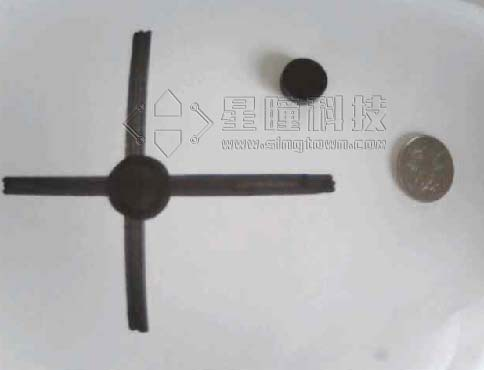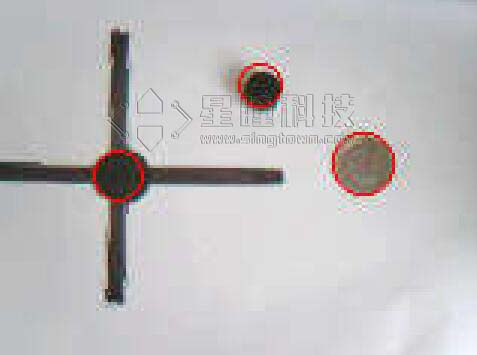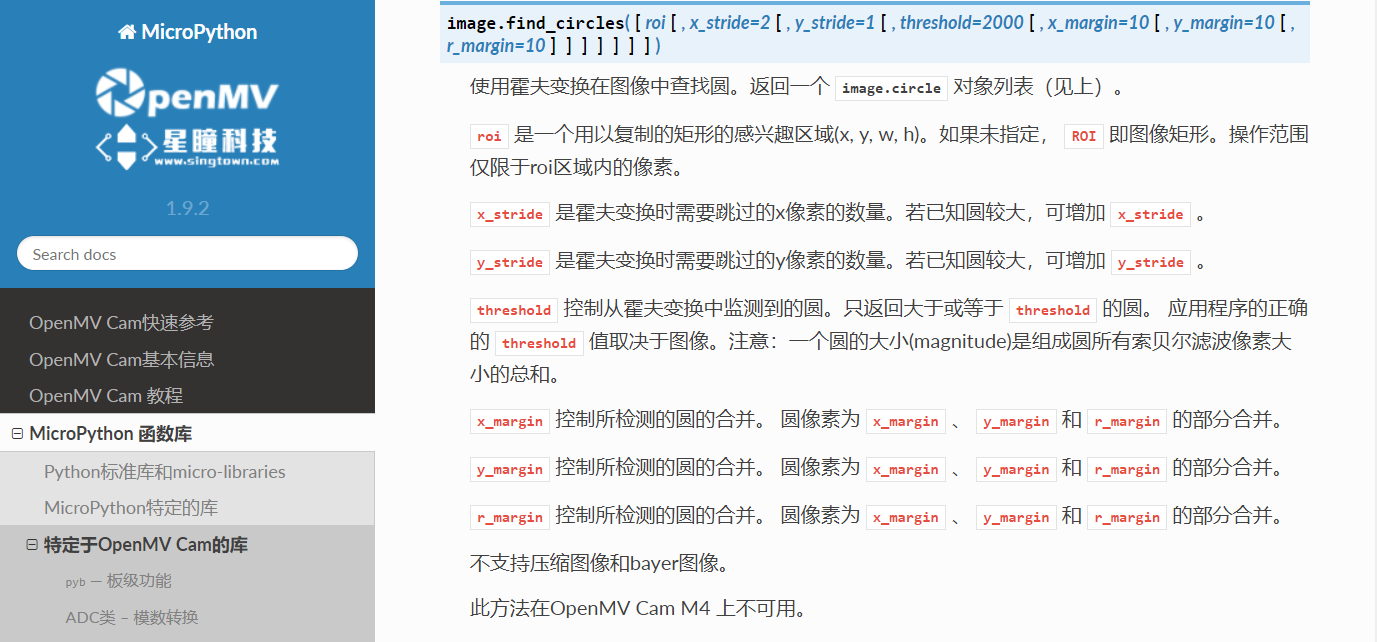# 例程讲解-09-find_circles识别圆

##### 视频教程21 - 追其他物体的小车：https://singtown.com/learn/50041/

``````# 圆形检测例程
#
# 这个例子展示了如何用Hough变换在图像中找到圆。
# https://en.wikipedia.org/wiki/Circle_Hough_Transform
#
# 请注意，find_circles（）方法将只能找到完全在图像内部的圆。圈子之外的
# 图像/ roi被忽略...

import sensor, image, time

sensor.reset()
sensor.set_pixformat(sensor.RGB565) # 灰度更快
sensor.set_framesize(sensor.QQVGA)
sensor.skip_frames(time = 2000)
clock = time.clock()

while(True):
clock.tick()

#lens_corr(1.8)畸变矫正
img = sensor.snapshot().lens_corr(1.8)

# Circle对象有四个值: x, y, r (半径), 和 magnitude。
# magnitude是检测圆的强度。越高越好

# roi 是一个用以复制的矩形的感兴趣区域(x, y, w, h)。如果未指定，
# ROI 即图像矩形。操作范围仅限于roi区域内的像素。

# x_stride 是霍夫变换时需要跳过的x像素的数量。若已知圆较大，可增加
# x_stride 。

# y_stride 是霍夫变换时需要跳过的y像素的数量。若已知直线较大，可增加
# y_stride 。

# threshold 控制从霍夫变换中监测到的圆。只返回大于或等于阈值的圆。
# 应用程序的阈值正确值取决于图像。注意：一条圆的大小是组成圆所有
# 索贝尔滤波像素大小的总和。

# x_margin 控制所检测的圆的合并。 圆像素为 x_margin 、 y_margin 和
# r_margin的部分合并。

# y_margin 控制所检测的圆的合并。 圆像素为 x_margin 、 y_margin 和
# r_margin 的部分合并。

# r_margin 控制所检测的圆的合并。 圆像素为 x_margin 、 y_margin 和
# r_margin 的部分合并。

# r_min，r_max和r_step控制测试圆的半径。
# 缩小测试圆半径的数量可以大大提升性能。
# threshold = 3500比较合适。如果视野中检测到的圆过多，请增大阈值；
# 相反，如果视野中检测到的圆过少，请减少阈值。
for c in img.find_circles(threshold = 3500, x_margin = 10, y_margin = 10, r_margin = 10,r_min = 2, r_max = 100, r_step = 2):
img.draw_circle(c.x(), c.y(), c.r(), color = (255, 0, 0))
print(c)

print("FPS %f" % clock.fps())
``````